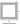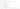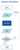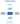Engineering designs are refined iteratively until an acceptable result is achieved. How about using the design synthesis methodology instead of the usual iterative method? What is the difference and how is synthesis superior compared to an iterative process?

In an iterative design process, a first cut design solution is laid out in CAD based on look, feel or experience and is mostly driven by space claim of various mechanisms. This first-cut design is taken through design validation calculations. Validation calculations are a set of design equations that best models the physical system. Iterating a design would then mean changing the input design variables deterministically to finally yield an acceptable result. If the problem is simple enough, it can also be driven to yield solution by optimization techniques over a single objective function. If the problem is not simple then the designer picks up a solution from among many that he “feels” is the best compromise.

Design synthesis process, in contrast is a way of math modeling of the design problem where design validation equations are now no longer written as sequential steps but as cross-influencing relationships of physical parameters. Solution is now a way of finding the best values for the parameters using optimization routines or even heuristic rules.

For instance, let’s take a uniform simply supported beam with a central load. If one would like to optimize the beam for weight for given maximum load and span and some maximum value of strength. The designer has the following variables to juggle with: material, grade, shape and section properties – width, height and thickness of section. There are at least five different variables. Weight is a function of section properties, material grade/strength.

DESIGN REQUIREMENTS:

Boom Length is given as 5 meters

Max Load to be lifted is 1500 Kgs.

Maximum principal stress should be less than 12 kgf/sqmm

Optimum cross section dimensions to have the least possible boom weight.#### DESIGN ITERATIONS

Let us see how to find the solution for this design using design iterations.

#### DESIGN PARAMETERS:

a, b and t are the section dimensions. Some arbitrary value for each of them is assumed and used for calculation.

#### CALCULATIONSAn arbitrary value for a, b and t is assumed and the above mentioned calculations are performed. This set of calculations are to be performed for every new value of a,b and t .

#### DESIGN GOALS:

To find the least possible boom weight for a given set of values for a, b and t within permissible limit of maximum principal stress.

#### ACCEPTANCE:

After the calculations are completed, we have the boom weight and stress values. Check if the maximum principal stress is within permissible limits for the selected material. If yes, the design is complete. If not, update design parameters i.e. a, b and t; and repeat the calculations. Find the boom weight and stresses check if they are acceptable or not.

#### DESIGN COMPLETE:

If the maximum principal stress is under permissible limits and the weight is supposed to be the least possible, then use the assumed a, b and t values as the section dimensions. The design is complete.

Different values of a, b and t are tried to achieve the design goals. This is a tiresome and non-optimal approach. After this process still it is unsure if the boom weight which is thought to be the least and optimum is actually the most optimum value.

#### DESIGN SYNTHESIS

Let us see how to find the solution to this design using design synthesis.#### DESIGN GOALS:

Reduced boom weight within given maximum principle stress. The required design parameters a, b, and t are included as open variables and will vary according to the relations provided.

#### RELATIONS AND EQUATIONS:

Equations and relations for boom weight, bending moment, bending stress, direct stress, and max principal stress are set up.

DESIGN CONSTRAINTS:

Maximum principal stress – Constraint is given so that the maximum principal stress is under the permissible limits.

#### DESIGN SYNTHESIS:

Boom weight is reduced with all the design relations and design constraints in place. By the end of this step we have optimized the design for least possible boom weight within the permissible stress limits.

#### DESIGN PARAMETERS:

Since all the relations are in place. Values of a, b and t for the least possible boom weight are attained.

#### DESIGN COMPLETE:

Cross section dimensions for the least possible boom weight and within the given design constraints is found. The design is complete.

Optimum boom weight is achieved in a process oriented approach.

#### Summary

In design iterations, the values of the cross section dimensions (design parameters) are assumed and a set of calculations are performed to find the boom weight and stresses (design goals). The maximum principal stress is checked if it is within the given permissible limits (acceptance). If scope to reduce the boom weight further is noticed then a new set of values for the cross section is assumed and the whole set of calculations are to be repeated until acceptable boom weight is achieved within the maximum principal stress. To achieve minimum boom weight in this kind of design problem is a very iterative and time consuming process. And it is still not guaranteed if the final weight and the shape of the cross section is the best possible value.

In design synthesis, the cross section dimensions are setup as open variables and the relations for boom weight and stresses are set up. The permissible limit for max principal stress is added as a design constraint. Taking the design relations, equations and constraints into consideration the design is synthesized. Boom weight is reduced until we have the least and optimized boom weight with acceptable cross section dimensions. The cross section dimensions are automatically attained as all the relations and constraints are already in place. We don’t have to iterate the design through the set of calculations repeatedly. So this way we achieve the minimum boom weight keeping the max principal stress within the permissible limits and also arriving at the cross section dimensions of the boom in an organized and process oriented approach.

As seen above, design synthesis encapsulates all the customer needs and working conditions into the problem at one go. The design iteration approach is more of a trial and error method and can take considerable time to reach a reasonable solution.

This way design synthesis is superior to design iterations as it is a process oriented approach to solving a design problem.